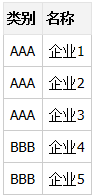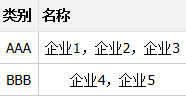# SQL SERVER 如何把1列多行数据 合并成一列显示 - 岁月寒风 - 博客园## 示例1 2 3 4 5 6 7 8 9 10 11 `select` `类别,` `    ``名称 = (` `        ``stuff(` `            ``(``select` `','` `+ 名称 ``from` `Table_A ``where` `类别 = A.类别 ``for` `xml path(``''``)),` `            ``1,` `            ``1,` `            ``''` `        ``)` `    ``)` `from` `Table_A ``as` `A ``group` `by` `类别`

for xml path(”)

stuff((select ‘,’ + ep_name from ep_detail where ep_classes = a.ep_classes for xml path(”)), 1, 1, ”)

### 觉得文章有用就打赏一下文章作者

#### 支付宝扫一扫打赏#### 微信扫一扫打赏• QQ咨询
• 回顶